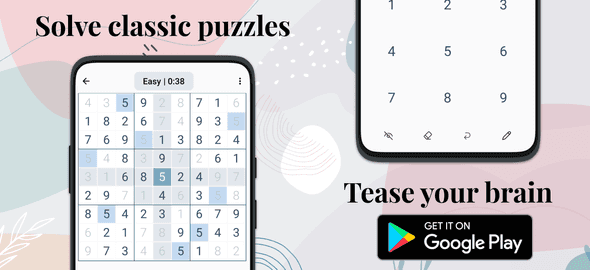### The 45 Rule in Sudoku: A Simple Strategy for Solving Puzzles

Sudoku is a popular puzzle game that challenges players to fill a 9x9 grid with digits from 1 to 9 while ensuring that each row, column, and 3x3 sub-grid contains all nine digits exactly once. While there are many strategies for solving Sudoku puzzles, one of the most basic and useful is the 45 rule.

## What is the 45 Rule in Sudoku?

The 45 rule is based on the fact that the digits in each row, column, and 3x3 sub-grid must add up to 45, since there are nine digits in total and each digit can only appear once in each region. For example, if you know that the sum of the digits in a row is 30, you can quickly deduce that the missing digits must add up to 15 (45 - 30) and use this information to fill in the remaining cells.

## How to Use the 45 Rule in Sudoku

To use the 45 rule, start by looking for rows, columns, or sub-grids with missing digits. Then, use the fact that the sum of the digits in each region must add up to 45 to deduce the missing digits. For example, if you have a row with the digits 1, 3, 4, 5, 7, and 9, you know that the sum of these digits is 29, so the missing digits must add up to 16 (45 - 29). From there, you can use logic and deduction to determine which digits are missing and where they belong in the row.

## Benefits of Using the 45 Rule in Sudoku

The 45 rule is a simple and intuitive strategy that can help you quickly solve Sudoku puzzles by narrowing down the possibilities and eliminating guesswork. By focusing on the sum of the digits in each region, you can avoid common mistakes and improve your accuracy and efficiency.

## Conclusion

The 45 rule is a powerful strategy for solving Sudoku puzzles that can help you make sense of complex patterns and quickly deduce missing digits. Whether you're a beginner or an experienced player, mastering the 45 rule can be a valuable tool in your Sudoku-solving arsenal. So, the next time you're stuck on a puzzle, try using the 45 rule and see how it can help you solve it in no time.